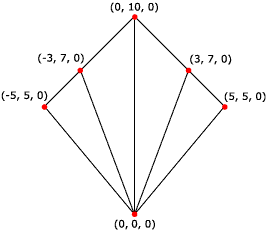# Triangle Fans (Direct3D 9)

A triangle fan is similar to a triangle strip, except that all the triangles share one vertex, as shown in the following illustration.The system uses vertices v2, v3, and v1 to draw the first triangle; v3, v4, and v1 to draw the second triangle; v4, v5, and v1 to draw the third triangle; and so on. When flat shading is enabled, the system shades the triangle with the color from its first vertex.

The following illustration depicts a rendered triangle fan.The following code shows how to create vertices for this triangle fan.

``````struct CUSTOMVERTEX
{
float x,y,z;
};

CUSTOMVERTEX Vertices[] =
{
{ 0.0, 0.0, 0.0},
{-5.0, 5.0, 0.0},
{-3.0,  7.0, 0.0},
{ 0.0, 10.0, 0.0},
{ 3.0,  7.0, 0.0},
{ 5.0,  5.0, 0.0},
};
``````

The code example below shows how to render this triangle fan in Direct3D 9 using IDirect3DDevice9::DrawPrimitive.

``````//
// It is assumed that d3dDevice is a valid
// pointer to a IDirect3DDevice9 interface.
//
d3dDevice->DrawPrimitive( D3DPT_TRIANGLEFAN, 0, 4 );
``````

Triangle fans are not supported in Direct3D 10 or later.

Primitives